# Github Profile :

https://github.com/DeepakKumar-1

# Graphs

A graph is simply a collection of nodes with edges between (some of) them.

• Graphs can be either directed or undirected. While directed edges are like a
one-way street, undirected edges are like a two-way street.
• The graph might consist of multiple isolated subgraphs.
• lf there is a path between every pair of vertices, it is called a “connected graph”
• The graph can also have cycles (or not). An “acyclic graph” is one without cycles.

### Graph Traversals

The two most common ways to search a graph are depth-first search(DFS) and breadth-first search(BFS).

• In depth-first search (DFS), we start at the root (or another arbitrarily selected node) and explore each branch completely before moving on to the next branch. That is, we go deep first (hence the name depth first search) before we go wide.

Time Complexity : `O(|E| + |V|)`
• In breadth-first search (BFS), we start at the root (or another arbitrarily selected node) and explore each neighbor before going on to any of their children. That is, we go wide (hence breadth-first search) before we go deep.

Time Complexity : `O(|E| + |V|)`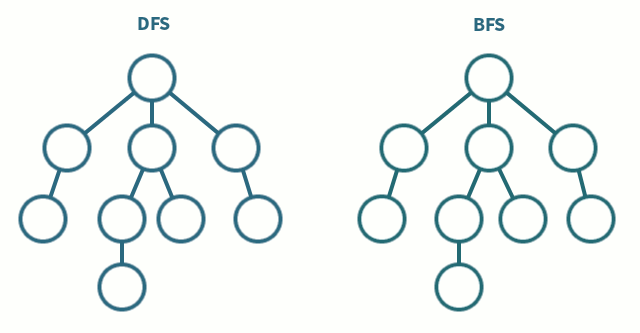### BFS Traversal Code

``````import java.util.*;

public class BFTraversal {
// BFTraversal of the Graph Similar to LEVEL ORDER Traversal of the Tree
public static void BFSTraversal(int [][] adjMatrix){
boolean [] visited = new boolean[adjMatrix.length];
}
public static void BFSTraversal(int [][] adjMatrix, boolean[] visited, int currentVertex){
// Create a Queue
return;
// Step 1 : Enqueue all the Neighbours of currentVertex
if(!visited[currentVertex]) {
visited[currentVertex] = true;
for (int i = 0; i < adjMatrix.length; i++) {
if (adjMatrix[currentVertex][i] == 1 && !visited[i]) {
visited[i] = true;
}
}
// Now Enqueue all the Elements from the Queue Until the Queue is Empty
while (!queue.isEmpty()) {
int element = queue.poll();
System.out.print(element + " ");
}
}
// Now Visit the Next Current Vertex

}

public static void main(String [] args){
Scanner sc = new Scanner(System.in);
// Take Number of Vertices and Edges from the USER
System.out.print(" Enter Number of Vertices: ");
int n = sc.nextInt();
System.out.print(" Enter Number of Edges: ");
int e = sc.nextInt();

int [][] adjMatrix = new int[n][n];
// Now Take All the Vertex Pairs
for(int i=0; i<e; i++){
int v1 = sc.nextInt();
int v2 = sc.nextInt();
}

// Now Display the Adjacency Matrix
System.out.println(Arrays.toString(row));
}

}
}
``````

### DFS Traversal Code

``````import java.util.*;

public class DFTraversal {
// Depth First Traversal in Graph
// Similar to PreOrder Traversal of TREE

public static void DFSTraversal(int [][]adjMatrix, int currentVertex, boolean []visited){
visited[currentVertex] = true;
System.out.print(currentVertex + " ");
if(adjMatrix[currentVertex][i] == 1 && visited[i] == false){
// So, i is Neighbour of Current Vertex
}
}
}

boolean [] visited = new boolean[adjMatrix.length];
for(int i=0; i< adjMatrix.length; i++) {
if(! visited[i]) {
System.out.println();
}
}
}

public static void main(String [] args){
// Take Number of Vertices and Edges from the USER
Scanner sc = new Scanner(System.in);
System.out.print(" Enter Number of Vertices: ");
int n = sc.nextInt();
System.out.print(" Enter Number of Edges: ");
int e = sc.nextInt();
int [][] adjMatrix = new int[n][n];
// Now take All the Edges with Initial and Final Vertices
for(int i=0; i<e; i++){
int v1 = sc.nextInt();
int v2 = sc.nextInt();
}
// Now Display the Adjacency Matrix
System.out.println(Arrays.toString(row));
}

// DFS Traversal
}
}
``````

### Kruskal’s Algorithm

• Kruskal’s algorithm finds a minimum spanning forest of an undirected edge-weighted graph.
• If the graph is connected, it finds a minimum spanning tree. (A minimum spanning tree of a connected graph is a subset of the edges that forms a tree that includes every vertex, where the sum of the weights of all the edges in the tree is minimized.
• For a disconnected graph, a minimum spanning forest is composed of a minimum spanning tree for each connected component.
• It is a greedy algorithm in graph theory as in each step it adds the next lowest-weight edge that will not form a cycle to the minimum spanning forest.

Time Complexity : `O(|E|log|V|)`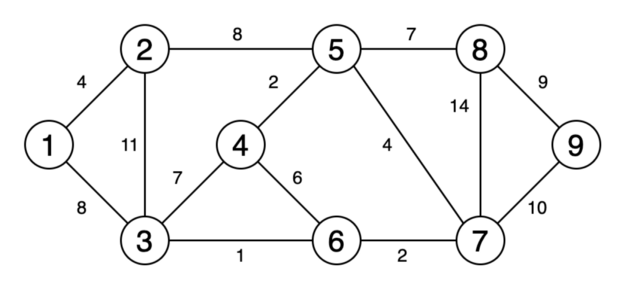### Kruskal’s Algorithm Code

``````import java.util.*;

class Edge implements Comparable<Edge>{
int v1;
int v2;
int weight;

Edge(int v1, int v2, int weight){
this.v1 = v1;
this.v2 = v2;
this.weight = weight;
}

@Override
public int compareTo(Edge o) {
return this.weight - o.weight;
}
}

public class KruskalsAlgorithm {

public static int findParent(int v, int []parent){
if(v == parent[v])
return v;
return findParent(parent[v], parent);
}

public static Edge[] KruskalAlgorithm(Edge[] edges, int n){
Arrays.sort(edges);
Edge []mst = new Edge[n - 1];
int [] parent = new int[n];
for(int j=0; j<n; j++){
parent[j] = j;
}

int count = 0;
int i=0;
while(count != n-1){
Edge currentEdge = edges[i++];
int v1Parent = findParent(currentEdge.v1, parent);
int v2Parent = findParent(currentEdge.v2, parent);
if(v1Parent != v2Parent){
// Including Current Edge
mst[count] = currentEdge;
count++;
parent[v1Parent] = v2Parent;
}
}
return mst;
}

public static void main(String [] args){
Scanner sc = new Scanner(System.in);
System.out.print("Enter Number of Vertices: ");
int n = sc.nextInt();
System.out.print("Enter Number of Edges: ");
int e = sc.nextInt();

Edge []edges = new Edge[e];
for(int i=0; i<e; i++){
int v1 = sc.nextInt();
int v2 = sc.nextInt();
int weight = sc.nextInt();
Edge edge = new Edge(v1, v2, weight);
edges[i] = edge;
;        }
Edge []mst = KruskalAlgorithm(edges, n);
for(int i=0; i< mst.length; i++){
if(mst[i].v1 < mst[i].v2){
System.out.println(mst[i].v1 + " " + mst[i].v2 + " " + mst[i].weight);
}else{
System.out.println(mst[i].v2 + " " + mst[i].v1 + " " + mst[i].weight);
}
}
}
}

// OUTPUT :
/*
Enter Number of Vertices: 8
Enter Number of Edges: 13
0 1 4
0 2 9
0 3 1
3 2 3
1 2 2
1 5 6
1 6 11
2 6 3
5 6 12
5 7 13
7 4 7
5 4 5
6 4 10

// Resultant MST

0 3 1
1 2 2
2 3 3
2 6 3
4 5 5
1 5 6
4 7 7
*/
``````

#### Prim’s Algorithm

• Prim’s algorithm (also known as Jarník’s algorithm) is a greedy algorithm that finds a minimum spanning tree for a weighted undirected graph.
• This means it finds a subset of the edges that forms a tree that includes every vertex, where the total weight of all the edges in the tree is minimized.
• The algorithm operates by building this tree one vertex at a time, from an arbitrary starting vertex, at each step adding the cheapest possible connection from the tree to another vertex.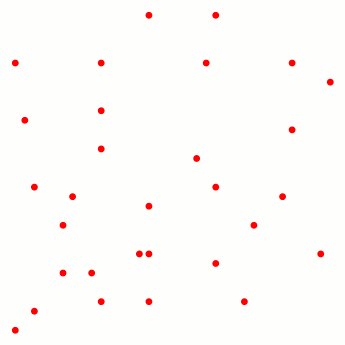### Prim’s Algorithm Code

``````import java.util.*;

public class PrimsAlgorithm {

public static void prims(int [][] adjMatrix){
boolean [] visited = new boolean[n];
int [] parent = new int[n];
int [] weight = new int[n];
// Source Vertex -> 0
parent = -1;
weight = 0;
for(int i=1; i<n; i++){
weight[i] = Integer.MAX_VALUE;
}
for(int i=0; i<n; i++){
int minVertex = findMinVertex(visited, weight);
visited[minVertex] = true;
// Explore Neighbours of Min Vertex
for(int j=0; j<n; j++){
// Update Value
parent[j] = minVertex;
}
}
}
}
//Print the MST
for(int i=1; i<n; i++){
if(i< parent[i]) {
System.out.println(i + " " + parent[i] + " " + weight[i]);
}else{
System.out.println(parent[i] + " " + i + " " + weight[i]);
}
}

}

private static int findMinVertex(boolean[] visited, int[] weight) {
int minVertex = -1;
for(int i=0; i< visited.length; i++){
if(!visited[i] && (minVertex == -1 || weight[i] < weight[minVertex])){
minVertex = i;
}
}
return minVertex;
}

public static void main(String [] args){
Scanner sc = new Scanner(System.in);
System.out.print("Enter Number of Vertices: ");
int n = sc.nextInt();
System.out.print("Enter Number of Edges: ");
int e = sc.nextInt();
int [][] adjMatrix = new int[n][n];
for(int i=0; i<e; i++){
int v1 = sc.nextInt();
int v2 = sc.nextInt();
int weight = sc.nextInt();
}
}
}
``````

#### Bellman-Ford Algorithm

• The Bellman–Ford algorithm is an algorithm that computes shortest paths from a single source vertex to all of the other vertices in a weighted digraph.
• Although it is slower than Dijkstra’s, it is more versatile, as it is capable of handling graphs in which some of the edge weights are
negative numbers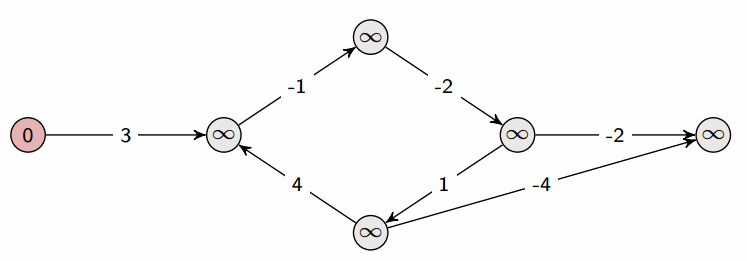#### Floyd-Warshall Algorithm

• Floyd-Warshall Algorithm is an algorithm for finding the shortest paths in a weighted graph with positive or negative edge weights, but
no negative cycles
• A single execution of the algorithm will find the lengths (summed weights) of the shortest paths between all pairs of nodes

#### Floyd-Warshall Algorithm Code

``````import java.util.*;
public class FloydWarshallAlgorithm {
final static int INF = 99999;
//    final static int INF = Integer.MAX_VALUE;

public static int [][] floyd_Warshall(int [][]graph) {
int v = graph.length;
int[][]dist = new int[v][v];
for(int i=0; i<v; i++){
for(int j=0; j<v; j++){
dist[i][j] = graph[i][j];
}
}
// Phases, in kth phase we include vertices (1,2, .... k) as intermediate Vertex
for (int k = 0; k < v; k++) {
// Iterate over 2D distance Matrix
for (int i = 0; i < v; i++) {
for (int j = 0; j < v; j++) {
// if Vertex k is included, and we can minimize the dist?
dist[i][j] = Math.min(dist[i][j], dist[i][k] + dist[k][j]);
}
}
}
//        for(int [] row: dist){
//            System.out.println(Arrays.toString(row));
//        }
return dist;
}

public static void main(String [] args) {

//        int[][] dist = {{0, 2, 1, INF, 3},
//                        {INF, 0,INF, 4, INF},
//                        {INF, 1, 0, INF, 1},
//                        {1, INF, 3, 0,5},
//                        {INF, INF, INF,INF, 0}};
int[][] dist = {{0, INF, -2, INF},
{4, 0, 3, INF},
{INF, INF, 0, 2},
{INF, -1, INF, 0}};
int [][]shortestPaths =  floyd_Warshall(dist);
for (int[] row : shortestPaths) {
System.out.println(Arrays.toString(row));
}

//        for(int i=0; i<shortestPaths.length; i++){
//            for(int j=0; j<shortestPaths.length; j++){
//                if(dist[i][j] == 99999){
//                    System.out.print("INF" + " ");
//                }else{
//                    System.out.print(dist[i][j] + " ");
//                }
//            }
//            System.out.println();
//        }
}
}
``````

#### Dijkstra’s Algorithm

• Dijkstra’s algorithm is very similar to Prim’s algorithm for minimum spanning tree. Like Prim’s MST, we generate a SPT (shortest path tree) with a given source as a root.
• We maintain two sets, one set contains vertices included in the shortest-path tree, other set includes vertices not yet included in the shortest-path tree.
• At every step of the algorithm, we find a vertex that is in the other set (set of not yet included) and has a minimum distance from the source.
• Dijkstra’s algorithm is used to find the shortest path from a single source vertex to all other vertices in the given graph.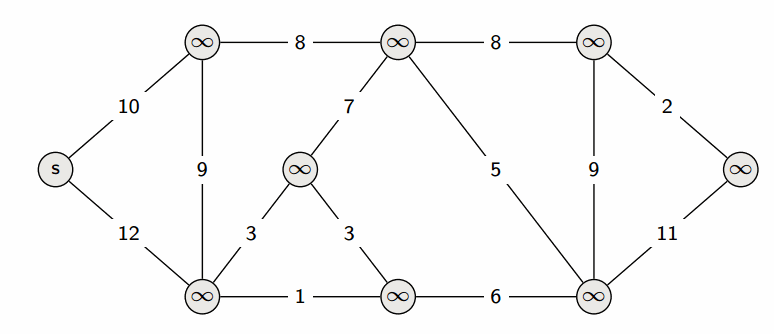### Dijkstra’s Algorithm Code

``````import java.util.Arrays;
import java.util.Scanner;

public class DijkstrasAlgorithm {

public static void dijkstra(int [][] adjMatrix){
boolean [] visited = new boolean[n];
int [] distance = new int[n];
distance = 0;
Arrays.fill(distance, 1, distance.length, Integer.MAX_VALUE);
for(int i=0; i<n-1; i++){
int minVertex = findMinVertex(visited, distance);
// mark minimum Vertex as True and Explore the Neighbours
visited[minVertex] = true;
for(int j=0; j<n; j++){
// unvisited Neighbour of minVertex
// Calculate distance to Reach j from source via MinVertex
int newDistance = distance[minVertex] + adjMatrix[minVertex][j];
if(newDistance < distance[j]){
distance[j] = newDistance;
}
}
}
}
// Printing Distance Values for All Vertices
for(int i=0; i<n; i++) {
System.out.println(i + " " + distance[i]);
}
}

private static int findMinVertex(boolean[] visited, int[] distance) {
int minVertex = -1;
for(int i=0; i<visited.length; i++){
if(!visited[i] && (minVertex == -1 || (distance[i] < distance[minVertex]))){
minVertex = i;
}
}
return minVertex;
}

public static void main(String [] args){
Scanner sc = new Scanner(System.in);
// take Number of Vertices and Edges from the USER
System.out.print("Enter Number of Vertices: ");
int n = sc.nextInt();
System.out.print("Enter Number of Edges: ");
int e = sc.nextInt();
// Create an Adjacency Matrix of size n*n
int [][] adjMatrix = new int[n][n];
for(int i=0; i<e; i++){
// take Initial Final and Weight
int v1 = sc.nextInt();
int v2 = sc.nextInt();
int weight = sc.nextInt();
// adjMatrix[v2][v1] = weight; // Assuming that Graph is Undirected
}
}
}

/*
// OUTPUT
Enter Number of Vertices: 8
Enter Number of Edges: 16
0 2 2
2 0 5
2 3 4
0 3 7
6 2 6
2 6 3
6 3 3
0 1 8
1 5 16
4 5 5
7 5 2
4 7 8
7 6 5
6 4 4
3 4 9
4 0 4

0 0
1 8
2 2
3 6
4 9
5 14
6 5
7 17
*/

``````

### Kosaraju’s Algorithm

• DFS-based algorithm that can be used to find SCCs of a directed graph called the Kosaraju’s algorithm.
• The basic idea of this algorithm is to run DFS twice.
• The first DFS is done on the original directed graph and record the ‘post-order’ traversal of the vertices as in finding topological sort.
• The second DFS is done on the transpose of the original directed graph using the ‘post-order’ ordering found by the first DFS.
• This two passes of DFS is enough to find the SCCs of the directed graph

Time Complexity: `O(|V| + |E|)`

### Kosaraju’s Algorithm Code

``````import java.util.*;

// KosarajuAlgorithm for SCC
// Time Complexity : O(V+E)

public class KosarajuAlgorithm {
private static final Stack<Integer> stack = new Stack<>();

private static void dft(int [][]adjMatrix,int currentVertex, boolean []visited){
visited[currentVertex] = true;
for(int i=0; i < adjMatrix.length; i++){
}
}
stack.push(currentVertex);
//        System.out.println(stack);
}

private static int [][] transpose(int [][]adjMatrix){
for(int i=0; i<n; i++){
for(int j=0; j<n; j++){
}
}
return dist;
}

private static void kosaraju_Algorithm(int[][] adjMatrix) {
boolean [] visited = new boolean[adjMatrix.length];
for (int v=0; v< adjMatrix.length; v++) {
if(!visited[v]) {
}
}
ArrayList<Integer> list = new ArrayList<>();
while(!stack.isEmpty()){
int element = stack.pop();
}
System.out.println(list);
for(int [] row: dist){
System.out.println(Arrays.toString(row));
}
// Apply DFS again and Print the Connected Components
boolean [] visit = new boolean[dist.length];
System.out.println("Connected Components are: ");
for (int v : list) {
if (!visit[v]) {
dfTraversal(dist, v, visit);
System.out.println();
}
}
}

private static void dfTraversal(int [][]adjMatrix, int currentVertex, boolean []visited){
visited[currentVertex] = true;
System.out.print(currentVertex + " ");
for(int i=0; i < adjMatrix.length; i++){
}
}
}

public static void main(String []args){
Scanner sc = new Scanner(System.in);
System.out.print("Enter Number of Vertices: ");
int n = sc.nextInt();
System.out.print("Enter Number of Edges: ");
int e = sc.nextInt();
for(int i=0; i<e; i++){
int v1 = sc.nextInt();
int v2 = sc.nextInt();
}
}
}

// OUTPUT
/*
Enter Number of Vertices: 6
Enter Number of Edges: 7
0 1
1 2
2 1
1 3
3 4
4 5
5 4
[0, 1, 3, 4, 5, 2]
[0, 0, 0, 0, 0, 0]
[1, 0, 1, 0, 0, 0]
[0, 1, 0, 0, 0, 0]
[0, 1, 0, 0, 0, 0]
[0, 0, 0, 1, 0, 1]
[0, 0, 0, 0, 1, 0]
Connected Components are:
0
1 2
3
4 5

Enter Number of Vertices: 5
Enter Number of Edges: 5
0 1
1 2
2 0
1 3
4 3
[4, 0, 1, 3, 2]
[0, 0, 1, 0, 0]
[1, 0, 0, 0, 0]
[0, 1, 0, 0, 0]
[0, 1, 0, 0, 1]
[0, 0, 0, 0, 0]
Connected Components are:
4
0 2 1
3
*/
``````

# Adjacency Matrix Representation of Graph

``````import java.util.Arrays;
import java.util.Scanner;
public static void main(String [] args){
// Take Number of Vertices and Edges from the USER
Scanner sc = new Scanner(System.in);
System.out.print("Enter Number of Vertices: ");
int n = sc.nextInt();
//Take Number of Edges from the USER
System.out.print("Enter Number of Edges: ");
int e = sc.nextInt();
int [][] adjMatrix = new int[n][n];
// Now Take Edges from the USER
for(int i=0; i<e; i++){
int v1 = sc.nextInt();
int v2 = sc.nextInt();
adjMatrix[v2][v1] = 1; // Assuming that Graph is Undirected
}
// Now Display the Resultant Adjacency Matrix